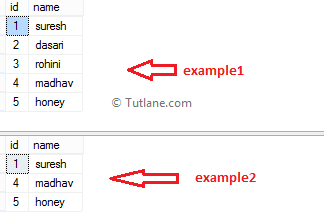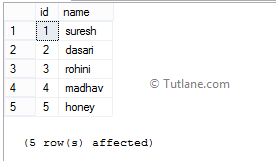# SQL Any Operator

In SQL, Any operator is used to return values that match with any value in a single column set of values. It’s like an OR operator, and it will compare a value against any value in the defined column.

To check any operator in SQL with examples, execute the following script to create a new table in the SQL database.

create table example1(id int, name varchar(50))
insert into example1 values(1,'suresh'),(2,'dasari'),(3,'rohini'),(4,'madhav'),(5,'honey')

create table example2(id int, name varchar(50))
insert into example2 values(1,'suresh'),(4,'madhav'),(5,'honey')

select * from example1
select * from example2

Once we execute the above script, the new table’s example1 and example2 will create and return the following result.Our tables are ready to check how ANY operator will work in SQL with examples, then execute the following queries.

## SQL Any Operator Example

Following is the example of using any operator in SQL.

SELECT id,name FROM example1 WHERE id = ANY(SELECT Id FROM example2)

When we execute the above SQL query, we will get a SQL any operator result like shown below.In the above query, the “id” column in the example1 table will compare with all id's in the example2 table.

(example1:id1 = example2:id1)
OR (example1:id1 = example2:id4)
OR (example1:id1 = example2:id5)

## SQL Any Operator Example2

Following is another example of using any operator in SQL.

SELECT id,name FROM example1 where id >= ANY(SELECT Id FROM example2)

When we execute the above query, we will get the result below.